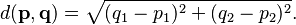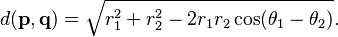# Euclidean distance facts for kids

Kids Encyclopedia Facts

In Euclidean geometry, the Euclidean distance is the usual distance between two points p and q. This distance is measured as a line segment. The Pythagorean theorem can be used to calculate this distance.

## Euclidean distance on the plane

In the Euclidean plane, if p = (p1p2) and q = (q1q2) then the distance is given by$d(\mathbf{p},\mathbf{q})=\sqrt{(q_1-p_1)^2 + (q_2-p_2)^2}.$

This is equivalent to the Pythagorean theorem, where legs are differences between respective coordinates of the points, and hypotenuse is the distance.

Alternatively, if the polar coordinates of the point p are (r1, θ1) and those of q are (r2, θ2), then the distance between the points is$d(\mathbf{p},\mathbf{q})=\sqrt{r_1^2 + r_2^2 - 2 r_1 r_2 \cos(\theta_1 - \theta_2)}.$

## Images for kidsEuclidean distance Facts for Kids. Kiddle Encyclopedia.